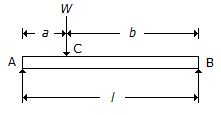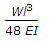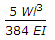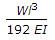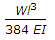# Mechanical Engineering - Strength of Materials

Exercise : Strength of Materials - Section 1
21.
In the torsion equation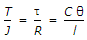the term J/R is called
shear modulus
section modulus
polar modulus
none of these
Explanation:
No answer description is available. Let's discuss.

22.
Strain rosettes are used to
measure shear strain
measure linear strain
measure volumetric strain
relieve strain
Explanation:
No answer description is available. Let's discuss.

23.
The torque transmitted by a solid shaft of diameter (D) is (where τ = Maximum allowable shear stress)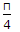x τ x D3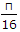x τ x D3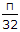x τ x D3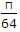x τ x D3
Explanation:
No answer description is available. Let's discuss.

24.
When a rectangular beam is loaded transversely, the maximum compressive stress is developed on the
top layer
bottom layer
neutral axis
every cross-section